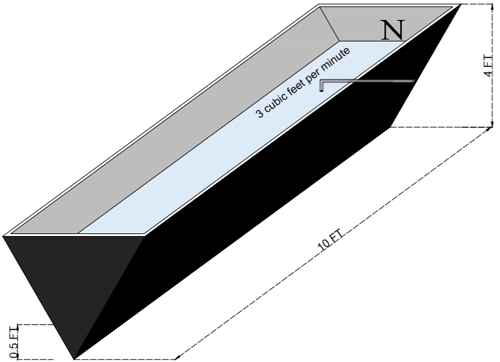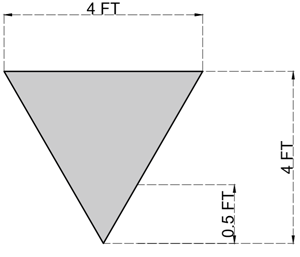### Math Notes

Subjects

#### Differential Calculus Solutions

##### Topics || Problems

A triangular trough is 10ft long 4ft across the top and 4 feet deep. If water flows in at the rate of 30 cu. ft. per min., find how fast the surface rising when the water is 6 in. deep.

Solution:By ratio and proportion on the triangle, at any given height, the base is same as the height ($$b = h$$). Thus at a base of the triangle at $$h = 0.5 ft$$ is also $$0.5 ft$$

$$Vol = A_{triangle}(10)$$

$$Vol = \frac{1}{2}bh (10)$$

As the water increases, the value of b and h are also changing, thus, b and h are not constants.

$$Vol = 5 h^2$$, Since $$b = h$$

$$\frac{dV}{dt} = 10 h \frac{dh}{dt}$$

At h =0.5 ft

$$3 = 10 (0.5) \frac{dh}{dt}$$

$$\frac{dh}{dt} = 0.6 \text{ft per min}$$

Thus the surface is rising at 0.6 feet per minute.